# Machine learning with missing values¶

Here we use simulated data to understanding the fundamentals of statistical learning with missing values.

This notebook reveals why a HistGradientBoostingRegressor ( `sklearn.ensemble.HistGradientBoostingRegressor` ) is a good choice to predict with missing values.

We use simulations to control the missing-value mechanism, and inspect it’s impact on predictive models. In particular, standard imputation procedures can reconstruct missing values without distortion only if the data is missing at random.

A good introduction to the mathematics behind this notebook can be found in https://arxiv.org/abs/1902.06931

## The fully-observed data: a toy regression problem¶

We consider a simple regression problem where X (the data) is bivariate gaussian, and y (the prediction target) is a linear function of the first coordinate, with noise.

### The data-generating mechanism¶

```import numpy as np

def generate_without_missing_values(n_samples, rng=42):
mean = [0, 0]
cov = [[1, 0.9], [0.9, 1]]
if not isinstance(rng, np.random.RandomState):
rng = np.random.RandomState(rng)
X = rng.multivariate_normal(mean, cov, size=n_samples)

epsilon = 0.1 * rng.randn(n_samples)
y = X[:, 0] + epsilon

return X, y
```

A quick plot reveals what the data looks like

```import matplotlib.pyplot as plt
plt.rcParams['figure.figsize'] = (5, 4) # Smaller default figure size

plt.figure()
X_full, y_full = generate_without_missing_values(1000)
plt.scatter(X_full[:, 0], X_full[:, 1], c=y_full)
plt.colorbar(label='y')
```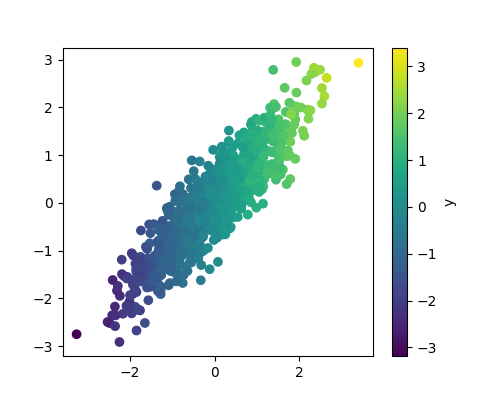```<matplotlib.colorbar.Colorbar object at 0x7fd79d08b8d0>
```

## Missing completely at random settings¶

We now consider missing completely at random settings (a special case of missing at random): the missingness is completely independent from the values.

### The missing-values mechanism¶

```def generate_mcar(n_samples, missing_rate=.5, rng=42):
X, y = generate_without_missing_values(n_samples, rng=rng)
if not isinstance(rng, np.random.RandomState):
rng = np.random.RandomState(rng)

M = rng.binomial(1, missing_rate, (n_samples, 2))

return X, y
```

A quick plot to look at the data

```X, y = generate_mcar(500)

plt.figure()
plt.scatter(X_full[:, 0], X_full[:, 1], color='.8', ec='.5', label='All data')
plt.colorbar(label='y')
plt.scatter(X[:, 0], X[:, 1], c=y, label='Fully observed')
plt.legend()
```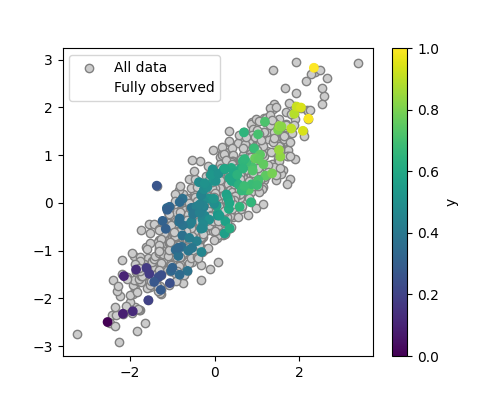```<matplotlib.legend.Legend object at 0x7fd79a391dd0>
```

We can see that the distribution of the fully-observed data is the same than that of the original data

### Conditional Imputation with the IterativeImputer¶

As the data is MAR (missing at random), an imputer can use the conditional dependencies between the observed and the missing values to impute the missing values.

We’ll use the IterativeImputer, a good imputer, but it needs to be enabled

```from sklearn.experimental import enable_iterative_imputer
from sklearn import impute
iterative_imputer = impute.IterativeImputer()
```

Let us try the imputer on the small data used to visualize

The imputation is learned by fitting the imputer on the data with missing values

```iterative_imputer.fit(X)
```
`IterativeImputer()`
In a Jupyter environment, please rerun this cell to show the HTML representation or trust the notebook.

The data are imputed with the transform method

```X_imputed = iterative_imputer.transform(X)
```

We can display the imputed data as our previous visualization

```plt.figure()
plt.scatter(X_full[:, 0], X_full[:, 1], color='.8', ec='.5',
label='All data', alpha=.5)
plt.scatter(X_imputed[:, 0], X_imputed[:, 1], c=y, marker='X',
label='Imputed')
plt.colorbar(label='y')
plt.legend()
```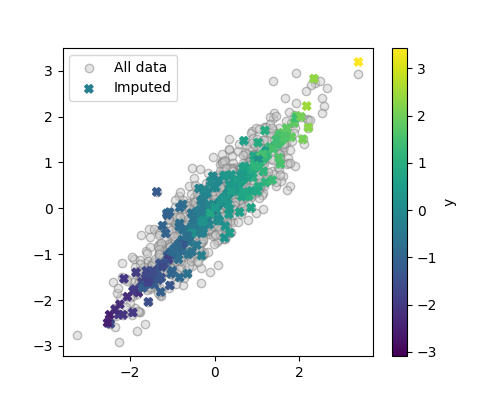```<matplotlib.legend.Legend object at 0x7fd79c1c1750>
```

We can see that the imputer did a fairly good job of recovering the data distribution

### Supervised learning: imputation and a linear model¶

Given that the relationship between the fully-observed X and y is a linear relationship, it seems natural to use a linear model for prediction. It must be adapted to missing values using imputation.

To use it in supervised setting, we will pipeline it with a linear model, using a ridge, which is a good default linear model

```from sklearn.pipeline import make_pipeline
from sklearn.linear_model import RidgeCV

iterative_and_ridge = make_pipeline(impute.IterativeImputer(), RidgeCV())
```

We can evaluate the model performance in a cross-validation loop (for better evaluation accuracy, we increase slightly the number of folds to 10)

```from sklearn import model_selection
scores_iterative_and_ridge = model_selection.cross_val_score(
iterative_and_ridge, X, y, cv=10)

scores_iterative_and_ridge
```
```array([0.61639853, 0.5814862 , 0.70136887, 0.64571923, 0.58785589,
0.79618649, 0.65278055, 0.8454113 , 0.81722841, 0.76948479])
```

Computational cost: One drawback of the IterativeImputer to keep in mind is that its computational cost can become prohibitive of large datasets (it has a bad computation scalability).

### Mean imputation: SimpleImputer¶

We can try a simple imputer: imputation by the mean

```mean_imputer = impute.SimpleImputer()
```

A quick visualization reveals a larger disortion of the distribution

```X_imputed = mean_imputer.fit_transform(X)
plt.figure()
plt.scatter(X_full[:, 0], X_full[:, 1], color='.8', ec='.5',
label='All data', alpha=.5)
plt.scatter(X_imputed[:, 0], X_imputed[:, 1], c=y, marker='X',
label='Imputed')
plt.colorbar(label='y')
```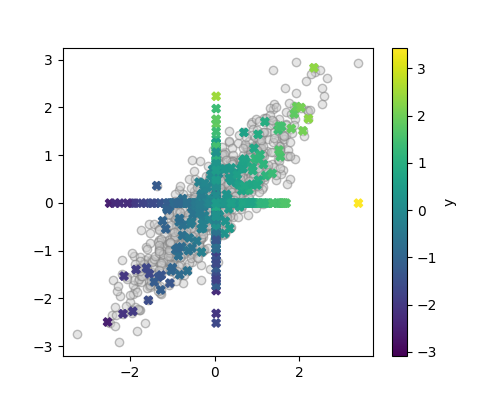```<matplotlib.colorbar.Colorbar object at 0x7fd757482a90>
```

Evaluating in prediction pipeline

```mean_and_ridge = make_pipeline(impute.SimpleImputer(), RidgeCV())
scores_mean_and_ridge = model_selection.cross_val_score(
mean_and_ridge, X, y, cv=10)

scores_mean_and_ridge
```
```array([0.58596256, 0.55215184, 0.61081314, 0.55282029, 0.54053836,
0.64325051, 0.60147921, 0.84188079, 0.68152965, 0.67441335])
```

### Supervised learning without imputation¶

The HistGradientBoosting models are based on trees, which can be adapted to model directly missing values

```from sklearn.experimental import enable_hist_gradient_boosting

```
```/home/varoquau/dev/scikit-learn/sklearn/experimental/enable_hist_gradient_boosting.py:15: UserWarning: Since version 1.0, it is not needed to import enable_hist_gradient_boosting anymore. HistGradientBoostingClassifier and HistGradientBoostingRegressor are now stable and can be normally imported from sklearn.ensemble.
warnings.warn(

array([0.61170698, 0.56911116, 0.635685  , 0.62752111, 0.58094569,
0.71569692, 0.62359162, 0.83829684, 0.81283438, 0.74080482])
```

### Recap: which pipeline predicts well on our small data?¶

Let’s plot the scores to see things better

```import pandas as pd
import seaborn as sns

scores = pd.DataFrame({'Mean imputation + Ridge': scores_mean_and_ridge,
'IterativeImputer + Ridge': scores_iterative_and_ridge,
})

sns.boxplot(data=scores, orient='h')
plt.title('Prediction accuracy\n linear and small data\n'
'Missing Completely at Random')
plt.tight_layout()
```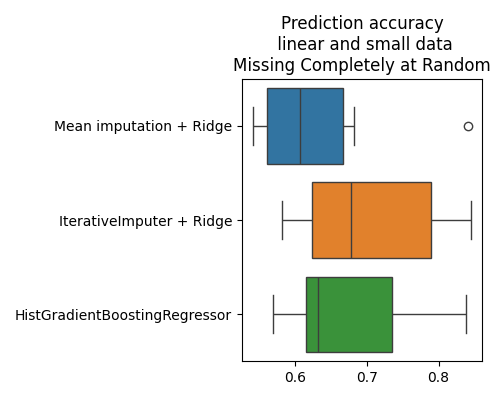Not much difference with the more sophisticated imputer. A more thorough analysis would be necessary, with more cross-validation runs.

### Prediction performance with large datasets¶

Let us compare models in regimes where there is plenty of data

```X, y = generate_mcar(n_samples=20000)
```

Iterative imputation and linear model

```scores_iterative_and_ridge= model_selection.cross_val_score(
iterative_and_ridge, X, y, cv=10)
```

Mean imputation and linear model

```scores_mean_and_ridge = model_selection.cross_val_score(
mean_and_ridge, X, y, cv=10)
```

And now the HistGradientBoostingRegressor, which does not need imputation

```score_hist_gradient_boosting = model_selection.cross_val_score(
```

We plot the results

```scores = pd.DataFrame({'Mean imputation + Ridge': scores_mean_and_ridge,
'IterativeImputer + Ridge': scores_iterative_and_ridge,
})

sns.boxplot(data=scores, orient='h')
plt.title('Prediction accuracy\n linear and large data\n'
'Missing Completely at Random')
plt.tight_layout()
```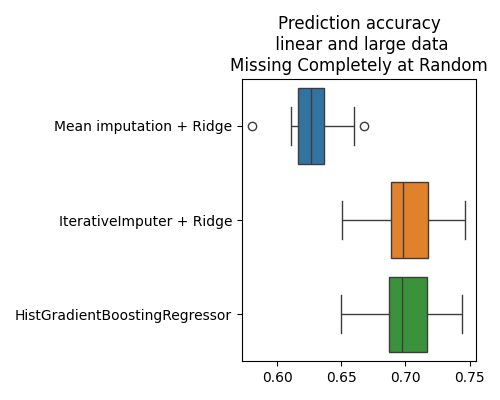When there is a reasonnable amout of data, the HistGradientBoostingRegressor is the best strategy even for a linear data-generating mechanism, in MAR settings, which are settings favorable to imputation + linear model .

## Missing not at random: censoring¶

We now consider missing not at random settings, in particular self-masking or censoring, where large values are more likely to be missing.

### The missing-values mechanism¶

```def generate_censored(n_samples, missing_rate=.4, rng=42):
X, y = generate_without_missing_values(n_samples, rng=rng)
if not isinstance(rng, np.random.RandomState):
rng = np.random.RandomState(rng)

B = rng.binomial(1, 2 * missing_rate, (n_samples, 2))
M = (X > 0.5) * B

return X, y
```

A quick plot to look at the data

```X, y = generate_censored(500, missing_rate=.4)

plt.figure()
plt.scatter(X_full[:, 0], X_full[:, 1], color='.8', ec='.5',
label='All data')
plt.colorbar(label='y')
plt.scatter(X[:, 0], X[:, 1], c=y, label='Fully observed')
plt.legend()
```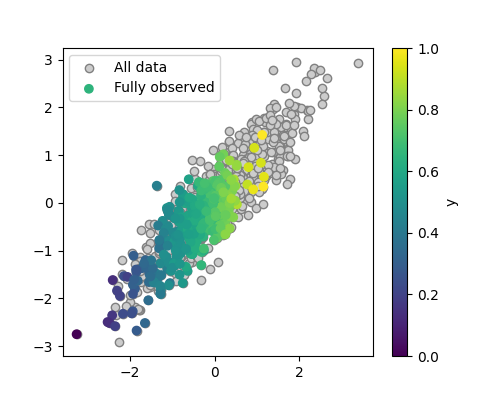```<matplotlib.legend.Legend object at 0x7fd754254e50>
```

Here the full-observed data does not reflect well at all the distribution of all the data

### Imputation fails to recover the distribution¶

With MNAR data, off-the-shelf imputation methods do not recover the initial distribution:

```iterative_imputer = impute.IterativeImputer()
X_imputed = iterative_imputer.fit_transform(X)

plt.figure()
plt.scatter(X_full[:, 0], X_full[:, 1], color='.8', ec='.5',
label='All data', alpha=.5)
plt.scatter(X_imputed[:, 0], X_imputed[:, 1], c=y, marker='X',
label='Imputed')
plt.colorbar(label='y')
plt.legend()
```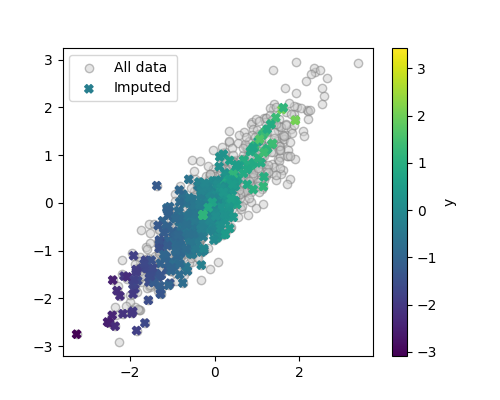```<matplotlib.legend.Legend object at 0x7fd7542d1c10>
```

Recovering the initial data distribution would need much more mass on the right and the top of the figure. The imputed data is shifted to lower values than the original data.

Note also that as imputed values typically have lower X values than their full-observed counterparts, the association between X and y is also distorted. This is visible as the imputed values appear as lighter diagonal lines.

An important consequence is that the link between imputed X and y is no longer linear, although the original data-generating mechanism is linear . For this reason, it is often a good idea to use non-linear learners in the presence of missing values.

### Predictive pipelines¶

Let us now evaluate predictive pipelines

```scores = dict()

# Iterative imputation and linear model
scores['IterativeImputer + Ridge'] = model_selection.cross_val_score(
iterative_and_ridge, X, y, cv=10)

# Mean imputation and linear model
scores['Mean imputation + Ridge'] = model_selection.cross_val_score(
mean_and_ridge, X, y, cv=10)

# IterativeImputer and non-linear model
iterative_and_gb = make_pipeline(impute.SimpleImputer(),
iterative_and_gb, X, y, cv=10)

# Mean imputation and non-linear model
mean_and_gb = make_pipeline(impute.SimpleImputer(),
mean_and_gb, X, y, cv=10)

# And now the HistGradientBoostingRegressor, whithout imputation

# We plot the results
sns.boxplot(data=pd.DataFrame(scores), orient='h')
plt.title('Prediction accuracy\n linear and small data\n'
'Missing not at Random')
plt.tight_layout()
```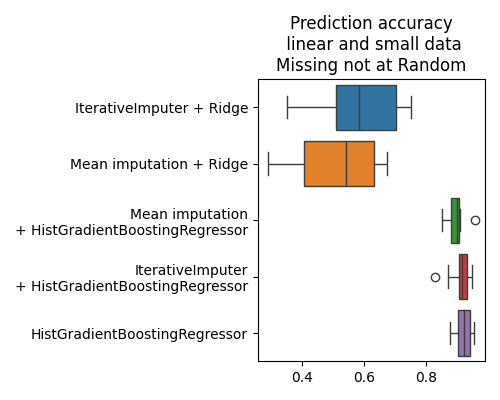We can see that the imputation is not the most important step of the pipeline , rather what is important is to use a powerful model. Here there is information in missingness (if a value is missing, it is large), information that a model can use to predict better.

## Using a predictor for the fully-observed case¶

Let us go back to the “easy” case of the missing completely at random settings with plenty of data

```n_samples = 20000

X, y = generate_mcar(n_samples, missing_rate=.5)
```

Suppose we have been able to train a predictive model that works on fully-observed data:

```X_full, y_full = generate_without_missing_values(n_samples)
full_data_predictor.fit(X_full, y_full)

model_selection.cross_val_score(full_data_predictor, X_full, y_full)
```
```array([0.98934188, 0.9897668 , 0.98932951, 0.98916616, 0.98898611])
```

The cross validation reveals that the predictor achieves an excellent explained variance; it is a near-perfect predictor on fully observed data

Now we turn to data with missing values. Given that our data is MAR (missing at random), we will use imputation to build a completed data that looks like the full-observed data

```iterative_imputer = impute.IterativeImputer()
X_imputed = iterative_imputer.fit_transform(X)
```

The full data predictor can be used on the imputed data

```from sklearn import metrics
metrics.r2_score(y, full_data_predictor.predict(X_imputed))
```
```0.7011318399077224
```

This prediction is less good than on the full data, but this is expected, as missing values lead to a loss of information. We can compare it to a model trained to predict on data with missing values

```X_train, y_train = generate_mcar(n_samples, missing_rate=.5)
na_predictor.fit(X_train, y_train)

metrics.r2_score(y, na_predictor.predict(X))
```
```0.7036144866135492
```

Applying a model valid on the full data to imputed data work almost as well as a model trained for missing values. The small loss in performance is because the imputation is imperfect.

### When the data-generation is non linear¶

We now modify a bit the example above to consider the situation where y is a non-linear function of X

```X, y = generate_mcar(n_samples, missing_rate=.5)
y = y ** 2

# Train a predictive model that works on fully-observed data:
X_full, y_full = generate_without_missing_values(n_samples)
y_full = y_full ** 2
full_data_predictor.fit(X_full, y_full)

model_selection.cross_val_score(full_data_predictor, X_full, y_full)
```
```array([0.97018219, 0.96754963, 0.96600898, 0.96971664, 0.96795887])
```

Once again, we have a near-perfect predictor on fully-observed data

On data with missing values:

```iterative_imputer = impute.IterativeImputer()
X_imputed = iterative_imputer.fit_transform(X)

from sklearn import metrics
metrics.r2_score(y, full_data_predictor.predict(X_imputed))
```
```0.5330009632809711
```

The full-data predictor works much less well

Now we use a model trained to predict on data with missing values

```X_train, y_train = generate_mcar(n_samples, missing_rate=.5)
y_train = y_train ** 2
na_predictor.fit(X_train, y_train)

metrics.r2_score(y, na_predictor.predict(X))
```
```0.657124508956336
```

The model trained on data with missing values works significantly better than that was optimal for the fully-observed data.

Only for linear mechanism is the model on full data also optimal for perfectly imputed data. When the function linking X to y has curvature, this curvature turns uncertainty resulting from missingness into bias .

Total running time of the script: ( 0 minutes 10.502 seconds)

Gallery generated by Sphinx-Gallery